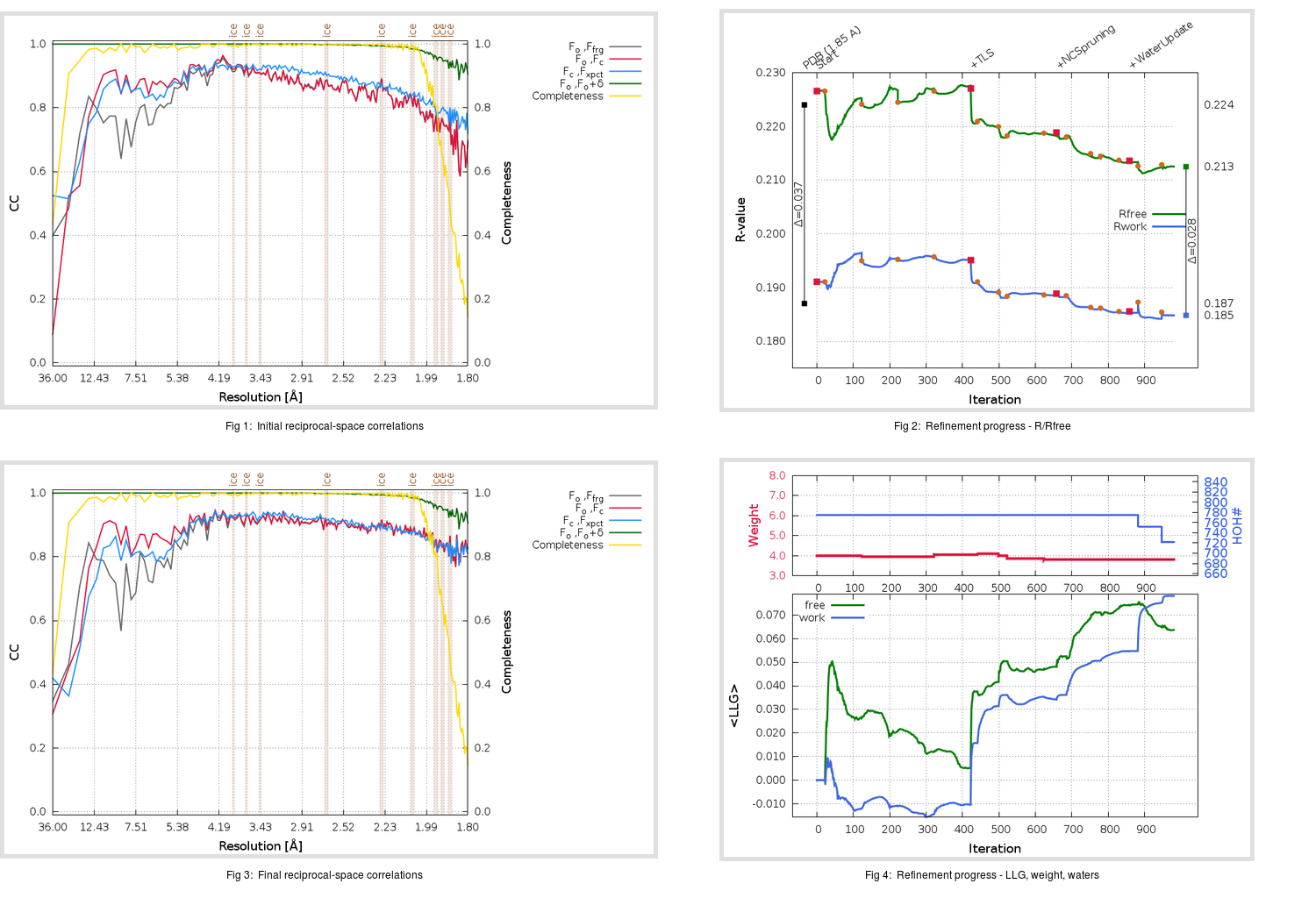Content:

```    Diffraction limits & principal axes of ellipsoid fitted to diffraction cut-off surface:
1.886         0.9636   0.0000   0.2673       0.999 a* + 0.053 c*
1.826         0.0000   1.0000   0.0000       b*
1.806        -0.2673   0.0000   0.9636      -0.202 a* + 0.979 c*
```

## Deposited

` `
 Date deposited Date data collection Resolution R, Rfree 20070920 20060228 1.85 0.1870 0.2240

Molprobity (CCP4 7.0 version) summary:

```Ramachandran outliers =   0.33 %
favored =  96.38 %
Rotamer outliers      =   3.62 %
C-beta deviations     =     0
Clashscore            =  12.53
RMS(bonds)            =   0.0061
RMS(angles)           =   1.52
MolProbity score      =   2.28
Resolution            =   1.85
R-work                =   0.1870
R-free                =   0.2240
```

```Number of waters      =   775

<B> (all atoms) =   28.54 ( sd =   10.14 ) for       5533 non-hydrogen atoms
<B>   (protein) =   26.58 ( sd =    8.83 ) for       4732 non-hydrogen atoms
<B>     (water) =   40.09 ( sd =    9.63 ) for        775 non-hydrogen atoms
<B>    (others) =   41.86 ( sd =    6.78 ) for         26 non-hydrogen atoms

B min/max       (all non-hydrogen atoms) =   12.26 /   71.59
B min/max   (protein non-hydrogen atoms) =   12.26 /   61.54
B min/max     (water non-hydrogen atoms) =   15.61 /   71.59
B min/max     (other non-hydrogen atoms) =   32.53 /   56.45
```

## BUSTER (re-)refinement

` `

Molprobity (CCP4 7.0 version) summary:

```Ramachandran outliers =   0.00 %
favored =  98.02 %
Rotamer outliers      =   4.57 %
C-beta deviations     =     0
Clashscore            =   3.50
RMS(bonds)            =   0.0112
RMS(angles)           =   1.54
MolProbity score      =   1.64
Resolution            =   1.80
R-work                =   0.1848
R-free                =   0.2125
```

```Number of waters      =   722

<B> (all atoms) =   29.42 ( sd =   12.14 ) for       5480 non-hydrogen atoms
<B>   (protein) =   27.22 ( sd =   10.39 ) for       4732 non-hydrogen atoms
<B>     (water) =   43.46 ( sd =   13.32 ) for        722 non-hydrogen atoms
<B>    (others) =   39.32 ( sd =    4.00 ) for         26 non-hydrogen atoms

B min/max       (all non-hydrogen atoms) =   13.56 /  115.18
B min/max   (protein non-hydrogen atoms) =   13.56 /   87.13
B min/max     (water non-hydrogen atoms) =   16.22 /  115.18
B min/max     (other non-hydrogen atoms) =   33.84 /   48.18
```

Refinement progression:Results:

` `
 File Remark 2Z9K_aB_refine.01_04_refine.pdb.gz exact refinement commands are in header 2Z9K_aB_refine.01_04_refine.mtz.gz including original deposited data and several re-refinement map coefficients 2Z9K_aB_refine.01_04_BUSTER_model.cif.gz including any non-standard compound restraints 2Z9K_aB_refine.01_04_BUSTER_refln.cif.gz